Next: 6.8 Activated Tunnelling From Up: 6 Quantum Diffusion II: Previous: 6.6 Experimental Results in

# 6.7 Numerical Evaluation of 1-Phonon Diffusion Theory

The direct evaluation of Eq. (6.20) by numerical methods was performed to generate the theoretical function used in fitting the temperature dependence of the Mu hop rate in terms of three parameters; the tunnelling bandwidth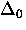, and dimensionless coupling parameters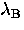and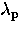. Since the phonon spectrum includes an arbitrary normalization that is folded into the coupling parameters, the overall normalization is arbitrary. However, the ratio of the coupling parameters tells us their relative strength, and determines the course of the temperature dependence.

Figure  6.29(a) shows an example of the integrand of Eq. (6.20), a function of time. The point to be made here is that the high temperature expansion of the general theory is clearly not appropriate for all values of the coupling constants. The structure in this function away from t=0 is due to phonons in the middle of the phonon spectrum; the highest frequencies contribute most to the region near t=0.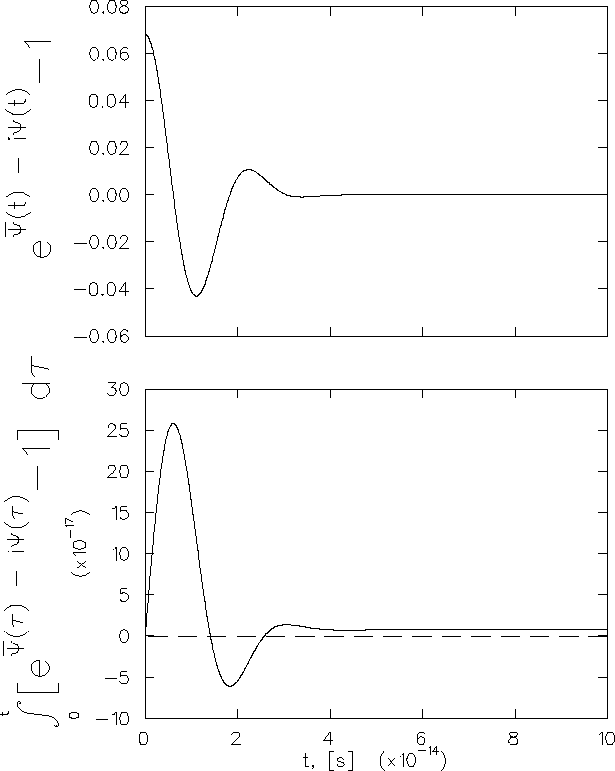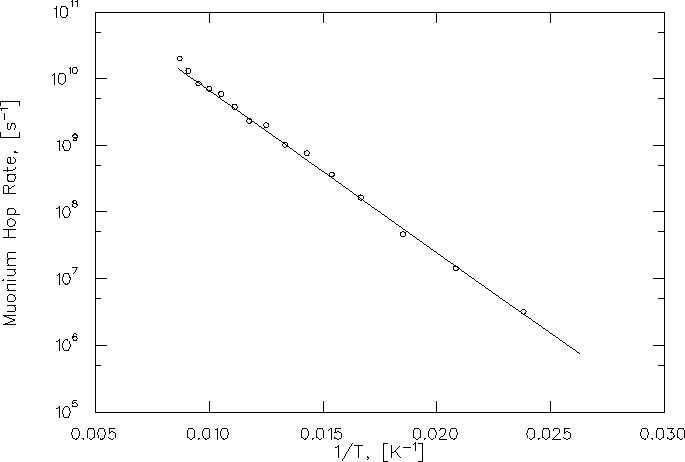Numerical evaluation of the integrals is made difficult due to the oscillatory nature of the integrand, which contains terms in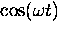while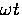ranges from zero to, in principle, infinity. However, the existence of an upper cut-off in the phonon spectrum at the Debye frequency allows one to carry out the integration to a time t such that an accurate estimate of the integral in the limit that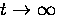can be made. (The program that performs this calculation is discussed in Apppendix B, complete with the code.) Fitting the data with hop rates calculated in this model gives the parameter values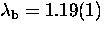and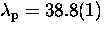.The resulting curve is also shown by the solid line in figure Fig. 6.30. The values of the parameters imply that the suppression of tunnelling due to the polaron self-trapping is overwhelmingly large in comparison to the benefit gained from barrier height fluctuations. A fit with the model of Flynn and Stoneham, with the hop rate given by Eq. (5.40) in which the barrier fluctuations do not enter, gives a similar fit with parameter values= 2.50(5)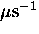and activation energy E = 0.0508(2) eV, also essentially indistinguishable from the full one-phonon theory.Next: 6.8 Activated Tunnelling From Up: 6 Quantum Diffusion II: Previous: 6.6 Experimental Results in